Helen在大都会机场工作，她的任务是安排每天的航班起飞时刻。今天一共有n架飞机将要起飞，第i架飞机将在第i分钟起飞。

Helen知道第i架飞机起飞时刻每延误一分钟机场所需支付的额外花费$c_i$是多少。帮助她找到额外花费最小的方案。

## 样例 #1

### 样例输入 #1

5 24 2 1 10 2

### 样例输出 #1

203 6 7 4 5

## 提示

$(3-1)·4+(6-2)·2+(7-3)·1+(4-4)·10+(5-5)·2=20$ burles.

## 题目分析

struct node{int t,val;//原计划起飞时间、赔偿金friend bool operator <(node a,node b){//每秒赔偿高的优先级高return a.val<b.val;}};

## 代码实现

#include <iostream>#include <cstdio>#include <queue>using namespace std;const int N=3e5+5;typedef long long ll;int n,k;int c[N];struct node{int t,val;friend bool operator <(node a,node b){//每秒赔偿高的优先级高return a.val<b.val;}};priority_queue<node> q;ll ans=0;int ord[N];int main(){cin>>n>>k;for(int i=1;i<=n;i++){cin>>c[i];q.push(node{i,c[i]});//飞机入队if(i<=k) continue;//飞机不得早于原定航班起飞 node cur=q.top();q.pop();//当前赔偿最高的优先起飞ans+=cur.val*(1LL*i-cur.t);//计算赔偿金额。注意相乘可能超intord[cur.t]=i;//记录飞机的起飞时间}for(int i=n+1;i<=n+k;i++){//安排后k架次的起飞顺序node cur=q.top();q.pop();//赔偿多的先起飞ans+=cur.val*(1LL*i-cur.t);ord[cur.t]=i;}cout<<ans<<endl;//输出总和for(int i=1;i<=n;i++){//输出各架次的起飞时间cout<<ord[i]<<" ";}return 0;}
]]>
<![CDATA[【题解】[CSP-J 2021] 插入排序]]> tag:https://www.algorithmnote.cn,2022-09-17:-ti-jie-csp-j2021-cha-ru-pai-xu 2022-09-17T10:15:09+08:00 2022-09-17T10:15:09+08:00 小鱼干 https://www.algorithmnote.cn [CSP-J 2021] 插入排序

## 题目描述

for (int i = 1; i <= n; i++)for (int j = i; j >= 2; j--)if (a[j] < a[j-1]) {int t = a[j-1];a[j-1] = a[j];a[j] = t;}

for i:=1 to n dofor j:=i downto 2 doif a[j]<a[j-1] thenbegint:=a[i];a[i]:=a[j];a[j]:=t;end;

H 老师给了一个长度为 $n$ 的数组 $a$，数组下标从 $1$ 开始，并且数组中的所有元素均为非负整数。小 Z 需要支持在数组 $a$ 上的 $Q$ 次操作，操作共两种，参数分别如下：

$1~x~v$：这是第一种操作，会将 $a$ 的第 $x$ 个元素，也就是 $a_x$ 的值，修改为 $v$。保证 $1 \le x \le n$$1 \le v \le 10^9$注意这种操作会改变数组的元素，修改得到的数组会被保留，也会影响后续的操作

$2~x$：这是第二种操作，假设 H 老师按照上面的伪代码$a$ 数组进行排序，你需要告诉 H 老师原来 $a$ 的第 $x$ 个元素，也就是 $a_x$，在排序后的新数组所处的位置。保证 $1 \le x \le n$注意这种操作不会改变数组的元素，排序后的数组不会被保留，也不会影响后续的操作

H 老师不喜欢过多的修改，所以他保证类型 $1$ 的操作次数不超过 $5000$

## 样例 #1

### 样例输入 #1

3 43 2 12 31 3 22 22 3

### 样例输出 #1

112

## 提示

【样例解释 #1】

【样例 #2】

【样例 #3】

【样例 #4】

【数据范围】

$1 \sim 4$$10$$10$
$5 \sim 9$$300$$300$
$10 \sim 13$$1500$$1500$
$14 \sim 16$$8000$$8000$保证所有输入的 $a_i,v$ 互不相同
$17 \sim 19$$8000$$8000$
$20 \sim 22$$8000$$2 \times 10^5$保证所有输入的 $a_i,v$ 互不相同
$23 \sim 25$$8000$$2 \times 10^5$

## 分析

### 暴力思路

bool cmp(node x,node y){if(x.v!=y.v) return x.v<y.v;return x.id<y.id;}### 优化1.0

scanf("%d",&x);    int cnt=0;    for(int j=1;j<=n;j++){        if(cmp(a[j],a[x])){            cnt++;        }    }    printf("%d\n",cnt+1);### 优化2.0

//site[x]:原序列a[x]在排好后的位置for(int i=1;i<=n;i++){    site[a[i].id]=i;}### 优化3.0

#include <iostream>#include <cstdio>#include <algorithm>using namespace std;int n,q;struct node{int id,val;}a;int site;//site[x] 原数列的第x个元素的当前位置/*3 2 12 3   => 11 3 2 =>3 2 22 2  12 3 2*/bool cmp(node x,node y){return x.val!=y.val?x.val<y.val:x.id<y.id;}//修改void upd(int x,int v){a[site[x]].val=v;//修改原数列中的值node key=a[site[x]];//往前挪删除原序列a[x] ，使得剩下n-1个元素为有序for(int i=site[x]+1;i<=n;i++){a[i-1]=a[i];}a[n]=key;//把修改后的值放到最后for(int i=n;i>1;i--){//插入 更新的值if(!cmp(a[i-1],a[i])){swap(a[i-1],a[i]);}}//更新sitefor(int i=1;i<=n;i++){site[a[i].id]=i;}}int main(){int k,x,v;scanf("%d%d",&n,&q);for(int i=1;i<=n;i++){scanf("%d",&a[i].val);a[i].id=i;}sort(a+1,a+n+1,cmp);//提前排序for(int i=1;i<=n;i++){//更新位置关系site[a[i].id]=i;}for(int i=1;i<=q;i++){scanf("%d",&k);if(k==2){scanf("%d",&x);printf("%d\n",site[x]);//输出位置}else if(k==1){scanf("%d%d",&x,&v);upd(x,v);//更新}}return 0;}]]>
<![CDATA[【题解】CSP-J2021_分糖果]]> tag:https://www.algorithmnote.cn,2022-09-15:-ti-jie-csp-j2021-fen-tang-guo 2022-09-15T17:03:50+08:00 2022-09-15T17:03:50+08:00 小鱼干 https://www.algorithmnote.cn [CSP-J 2021] 分糖果

## 样例 #1

### 样例输入 #1

7 16 23

### 样例输出 #1

6

## 样例 #2

### 样例输入 #2

10 14 18

### 样例输出 #2

8

## 样例 #3

### 样例输入 #3

见附件中的 candy/candy3.in。

### 样例输出 #3

见附件中的 candy/candy3.ans。

## 提示

【样例解释 #1】

$k = 20$ 块糖放入篮子里。

【样例解释 #2】

【数据范围】

$1$$2$$5$$5$
$2$$5$$10$$10$
$3$${10}^3$${10}^3$${10}^3$
$4$${10}^5$${10}^5$${10}^5$
$5$${10}^3$${10}^9$$0$
$6$${10}^3$${10}^9$${10}^3$
$7$${10}^5$${10}^9$${10}^5$
$8$${10}^9$${10}^9$${10}^9$
$9$${10}^9$${10}^9$${10}^9$
$10$${10}^9$${10}^9$${10}^9$

## 分析

#include <iostream>#include <cstdio>using namespace std;int main(){int n,l,r,ans=0;cin>>n>>l>>r;//输入小朋友的个数、糖果数量的下界和上界for(int i=l;i<=r;i++){//遍历l~rans=max(ans,i%n);}cout<<ans;return 0;}

$0、1、2、\cdots 、n-1、0、1、2、\cdots 、n-1、\cdots$

if(L/n==R/n){    cout<<R%n;}else{    cout<<n-1;}

## 实现代码

#include <iostream>#include <cstdio>using namespace std;int main(){int n,l,r;cin>>n>>l>>r;//输入小朋友的个数、糖果数量的下界和上界if(l/n==r/n){//包含余数中不含n-1cout<<r%n;}else{cout<<n-1;}return 0;}
]]>
<![CDATA[如何在洛谷写博客]]> tag:https://www.algorithmnote.cn,2022-09-03:ru-he-zai-luo-gu-xie-bo-ke 2022-09-03T23:22:44+08:00 2022-09-03T23:22:44+08:00 小鱼干 https://www.algorithmnote.cn 准备

​首先需要有一个能发布文章的账号。现阶段网络上有许多可以发布文章的地方，CSDN、博客园、简书、洛谷等都是可以免费发布文章的。

​其次是需要有写的素材。比如有一道经典的题，我们进行了认真的思考，可以写出思考的过程和实现方法；也可以整理所学的内容成为一篇文章。

## 发布在洛谷

1. step11. step2

点击“开通博客”1. step31. step4]]>
<![CDATA[【题解】糟糕的一天]]> tag:https://www.algorithmnote.cn,2022-05-27:-ti-jie--zao-gao-de-yi-tian 2022-05-27T23:32:58+08:00 2022-06-17T22:08:31+08:00 小鱼干 https://www.algorithmnote.cn 题面翻译

## 题目描述

Some of Farmer John’s N cows (1 ≤ N ≤ 80,000) are having a bad hair day! Since each cow is self-conscious about her messy hairstyle, FJ wants to count the number of other cows that can see the top of other cows’ heads.

Each cow i has a specified height hi (1 ≤ hi ≤ 1,000,000,000) and is standing in a line of cows all facing east (to the right in our diagrams). Therefore, cow i can see the tops of the heads of cows in front of her (namely cows i+1, i+2, and so on), for as long as these cows are strictly shorter than cow i.

Consider this example:

        --       --   -   --   -   -- - - - -- - - - - -1 2 3 4 5 6Cows facing right -->

Cow#1 can see the hairstyle of cows #2, 3, 4

Cow#2 can see no cow’s hairstyle

Cow#3 can see the hairstyle of cow #4

Cow#4 can see no cow’s hairstyle

Cow#5 can see the hairstyle of cow 6

Cow#6 can see no cows at all!

Let ci denote the number of cows whose hairstyle is visible from cow i; please compute the sum of c1 through cN.For this example, the desired is answer 3 + 0 + 1 + 0 + 1 + 0 = 5.

## 输入格式

Line 1: The number of cows, N.

Lines 2…N+1: Line i+1 contains a single integer that is the height of cow i.

## 输出格式

Line 1: A single integer that is the sum of c1 through cN.

## 样例 #1

### 样例输入 #1

610374122

### 样例输出 #1

5

## 题目分析

10 3 7 4 12 2

10： 3、 7、 4，能看到3头牛，之所以没有2，是因为v中间有个12，挡住了2。

3：一个都看不到。

7：4，能看到1头牛。

4：一个都看不到。

12：2，能看到1头牛。

2：一个都看不到。

while(!s.empty()&&s.top()<=x){    s.pop();}

## 代码实现

#include <iostream>#include <cstdio>#include <stack>using namespace std;const int N=8e4+5;typedef long long ll;stack<int> s;//定义栈int main(){int n,x;ll sum=0;//最终的总和cin>>n;for(int i=1;i<=n;i++){cin>>x;while(!s.empty()&&s.top()<=x){//不断将<=x的元素出栈s.pop();}sum+=s.size();//累加能看到x的元素个数s.push(x);//栈中元素呈单调减}cout<<sum;return 0;}

]]>
<![CDATA[最近公共祖先LCA（倍增思想）]]> tag:https://www.algorithmnote.cn,2022-05-18:zui-jin-gong-gong-zu-xian-lca-bei-zeng-si-xiang- 2022-05-18T16:48:23+08:00 2022-05-19T08:58:39+08:00 小鱼干 https://www.algorithmnote.cn 定义

## 算法

### 朴素算法

void dfs(int rt,int fr){//rt-当前节点 fr-rt的父结点//搜索，遍历树的结点，并记录深度信息    //dep[x] 结点x的深度 fa[x] 结点x的父结点for(int i=0;i<(int)G[rt].size();i++){int u=G[rt][i];if(u==fr) continue;//如果 遍历到rt的父结点，则跳过dep[u]=dep[rt]+1;        fa[u]=rt;//更新 rt为 u 的父结点dfs(u,rt);}}

int query_LCA(int x,int y){if(dep[x]<dep[y]) swap(x,y); //保证dep[x]>=dep[y]//1. 提到同一深度  将x往上提while(dep[x]!=dep[y]){x=fa[x];//往上提}//2. 同时往上提，直到重复while(x!=y){x=fa[x];y=fa[y];}return x;}

### 倍增算法

f[i][j] 为结点i的第$2^j$ 个父辈。for(int j=1;j<=Log[n];j++){    for(int i=1;i<=n;i++){        fa[i][j]=fa[fa[i][j-1]][j-1];    }}

$dev$看做二进制形式可被表达为 $2^{x_1} + 2^{x_2}+\cdots + 2^{x_k}$$x_i$ 代表$dev$二进制中为1的位数。那么我的x结点要提高y的高度只需要进过$k$(dev二进制的1的个数)步就可以。

int dev=dep[x]-dep[y];while(dev){    int k=lowBit(dev);    x=fa[x][Log[k]];    dev&=(dev-1);}

for(int i=Log[dep[x]];i>=0;i--){    if(fa[x][i]!=fa[y][i]){        x=fa[x][i];        y=fa[y][i];    }}return fa[x];

### 程序实现

#include <iostream>#include <cstdio>#include <vector>#include <cstring>using namespace std;const int N=5e5+5;vector<int>G[N];int n,m,s;int dep[N];int fa[N];//fa[x][k] x的2^k 辈父亲结点int Log[N];void dfs(int rt,int ff){//搜索，遍历树的结点并，并记录深度信息for(int i=0;i<(int)G[rt].size();i++){int u=G[rt][i];if(u==ff) continue;//如果 遍历到rt的父结点，则跳过dep[u]=dep[rt]+1;fa[u]=rt;//更新 rt为 u 的父结点dfs(u,rt);}}int lowBit(int x){return x&(-x);}int query_LCA(int x,int y){if(dep[x]<dep[y]) swap(x,y); //保证dep[x]>=dep[y]//1. 提到同一深度  将x往上提if(dep[x]!=dep[y]){//不同深度，则将x向上提至相同深度int hx=dep[x]-dep[y];while(hx){int k=lowBit(hx);x=fa[x][Log[k]];hx&=(hx-1);}}if(x==y) return x;//2. 同时往上提，直到重复for(int i=Log[dep[x]];i>=0;i--){if(fa[x][i]!=fa[y][i]){x=fa[x][i];y=fa[y][i];}}return fa[x];}int main(){int x,y,a,b;scanf("%d%d%d",&n,&m,&s);for(int i=1;i<n;i++){scanf("%d%d",&x,&y);G[x].push_back(y);G[y].push_back(x);}dep[s]=1;dfs(s,0);//预处理Log[]数组 ， Log[x]=log2(x)for(int i=2;i<=n;i++){Log[i]=Log[i/2]+1;}////预处理fa[x][y]= x的第2^y辈的结点for(int j=1;j<=Log[n];j++){for(int i=1;i<=n;i++){fa[i][j]=fa[fa[i][j-1]][j-1];}}for(int i=1;i<=m;i++){scanf("%d%d",&a,&b);printf("%d\n",query_LCA(a,b));}return 0;}
]]>
<![CDATA[【题解】玉蟾宫]]> tag:https://www.algorithmnote.cn,2022-05-12:-ti-jie--yu-chan-gong 2022-05-12T22:11:30+08:00 2022-05-18T16:48:41+08:00 小鱼干 https://www.algorithmnote.cn 题目背景

## 输入输出样例

5 6 R F F F F F F F F F F F R R R F F F F F F F F F F F F F F F

45

## 题目分析

up[x][y] 为从$(x,y)$ 位置向上符合条件的最长线段长度,即悬线长度。

L[x][y]为从$(x,y)$ 位置向左，悬线能平移的最长距离。

R[x][y]为从$(x,y)$ 位置向右，悬线能平移的最长距离。

## 代码实现

#include <iostream>#include <cstdio>using namespace std;const int N=1e3+5;int up[N][N],L[N][N],R[N][N];/*up[x][y] 从(x,y)开始，向上连续F的最大长度L[x][y] 从(x,y)开始，向左连续F的最大长度R[x][y] 从(x,y)开始，向右连续F的最大长度*/int a[N][N];int n,m;int main(){char c;cin>>n>>m;for(int i=1;i<=n;i++){for(int j=1;j<=m;j++){cin>>c;a[i][j]=(c=='F')?1:0;if(a[i][j]){//当(x,y)满足条件up[i][j]=up[i-1][j]+1;//更新悬线L[i][j]=L[i][j-1]+1;//更新从(i,j)向左的满足条件的最长长度}}for(int j=m;j>=1;j--){if(a[i][j]) R[i][j]=R[i][j+1]+1;//更新从(i,j)向右的满足条件的最长长度}}/*更新 L[][] 和 R[][] 为 从(x,y)想左/右的最大悬线平移距离L[i][j]=min(L[i][j],L[i-1][j])R[i][j]=min(R[i][j],LR[i-1][j])*/int maxs=0;for(int i=1;i<=n;i++){for(int j=1;j<=m;j++){if(i>=2&&a[i][j]&&a[i-1][j]){L[i][j]=min(L[i][j],L[i-1][j]);R[i][j]=min(R[i][j],R[i-1][j]);}maxs=max(maxs,up[i][j]*(L[i][j]+R[i][j]-1));//更新最大子矩阵面积}}cout<<3*maxs;return 0;}
]]>
<![CDATA[悬线法处理最大子矩阵问题]]> tag:https://www.algorithmnote.cn,2022-05-12:xuan-xian-fa-chu-li-zui-da-zi-ju-zhen-wen-ti 2022-05-12T21:27:10+08:00 2022-05-18T16:49:06+08:00 小鱼干 https://www.algorithmnote.cn 适用场景

## 悬线法思想及实现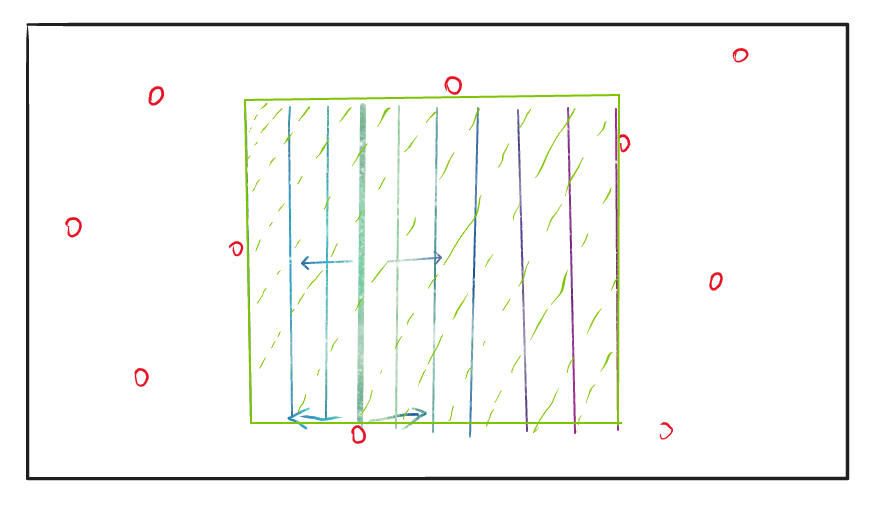up[x][y] 为从$(x,y)$ 位置向上符合条件的最长线段长度。

L[x][y]为从$(x,y)$ 位置向左符合条件的最长线段长度。

R[x][y]为从$(x,y)$ 位置向右符合条件的最长线段长度。

up[x][y]=up[x-1][y]+1;L[x][y]=L[x][y-1]+1;R[x][y]=R[x][y+1]+1;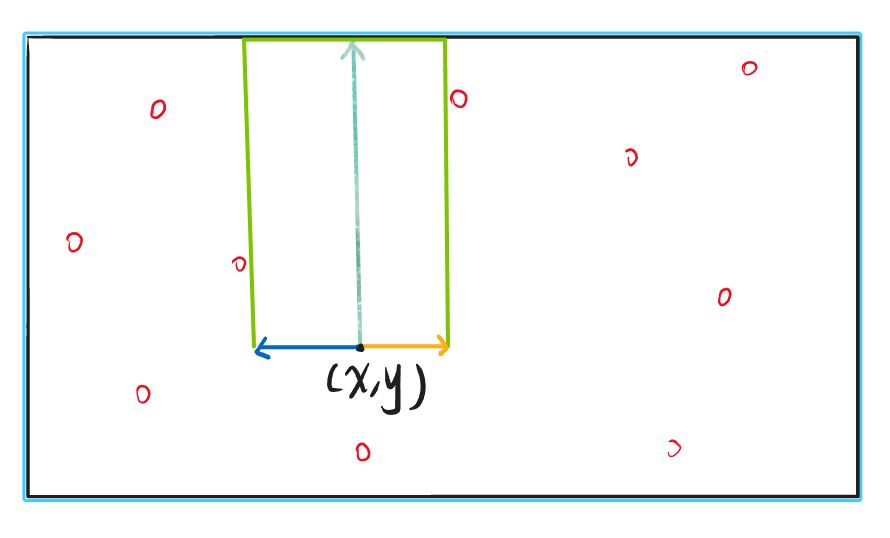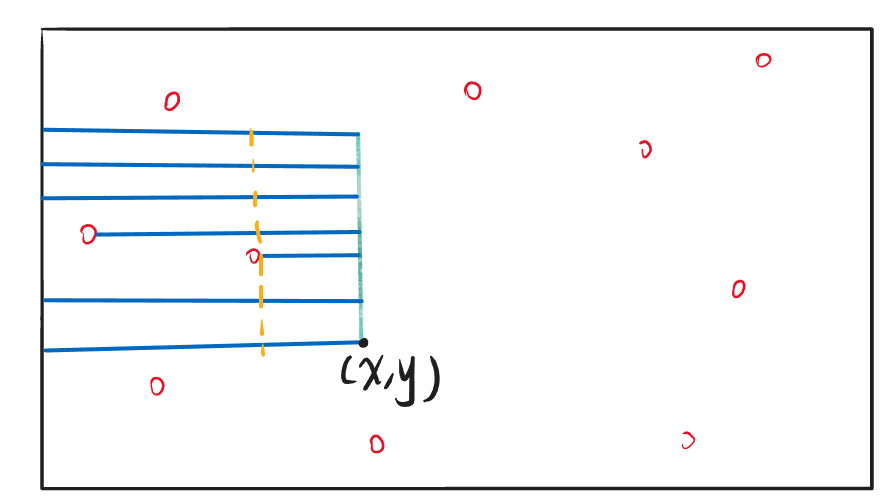$L[x][y]=min(L[x-1][y],L[x][y])$

$R[x][y]=min(R[x-1][y],R[x][y])$

for(int i=1;i<=n;i++){    for(int j=1;j<=m;j++){        if(i>=2 && a[i][j]与a[i-1][j]属于同一悬线){            L[i][j]=min(L[i-1][j],L[i][j]);            R[i][j]=min(R[i-1][j],R[i][j]);        }    }}

1. 从该点除法向上延伸的悬线长度
2. 从该点位置向左，悬线能平移的最长距离
3. 从该点位置向右，悬线能平移的最长距离

## 模板例题

]]>
<![CDATA[ST表算法与代码实现]]> tag:https://www.algorithmnote.cn,2022-05-12:st-biao-suan-fa-yu-dai-ma-shi-xian 2022-05-12T10:37:16+08:00 2022-05-12T10:40:44+08:00 小鱼干 https://www.algorithmnote.cn 前言

## ST表思想与原理

ST表的核心思想是倍增，设连续区域为$[L,R]$，若将连续区域分为左右两半，左半部分的最值为$max_{L}$,右半部分的最值为$max_R$ 。整个连续区域的最值就为左、右两个区域中最值的较大值也就是$max(max_L,max_R)$ 。思想如下图：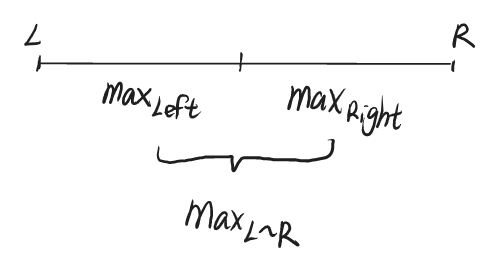f[i][j] 为从位置i开始的长为$2^j$ 区域内的最值。

$2^j=2^{j-1}+2^{j-1}$ ，我们将长度为$2^j$ 的区域均分为两段，分别长为$2^{j-1}$ 。那么整个长为$2^j$ 区域的最值就为左、右两段长为$2^{j-1}$区域最值的较大值。设区域从位置i开始，那么整个区域的最值可表达为f[i][j]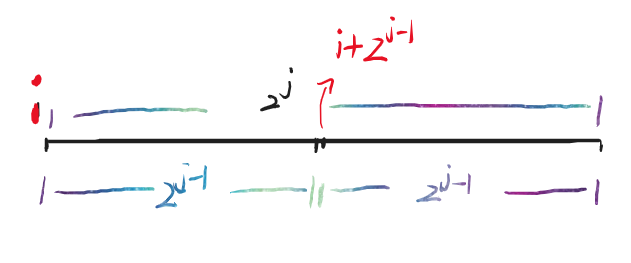### 预处理、维护过程

void stPrework(){//ST表预处理for(int i=1;i<=n;i++){//f[i][j]=从i开始，长为2^j区间内的最值f[i]=a[i];//长为1时的最值}for(int j=1;j<=Log[n];j++){//根据最长区间长度log[n]进行遍历for(int i=1;i+(1<<j)-1<=n;i++){//遍历开始位置if[i][j]=max(f[i][j-1],f[i+(1<<(j-1))][j-1]);//左边的最值与右边最值中较大者为整个区域的最值}}}

### 询问区域最值

$L\leq x \leq y \leq R$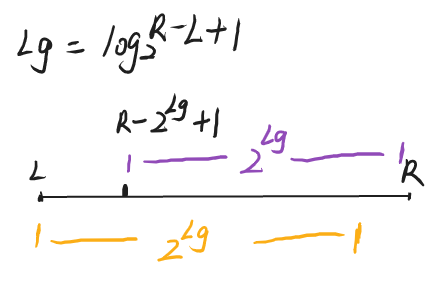int stQuery(int l,int r){//询问l~r区间的最值int Lg=Log[r-l+1];//计算l~r之间长度对应的log2值return max(f[l][Lg],f[r-(1<<Lg)+1][Lg]); }

## 总结

]]>
<![CDATA[【题解】平衡队列]]> tag:https://www.algorithmnote.cn,2022-05-11:-ti-jie--ping-heng-dui-lie 2022-05-11T23:22:27+08:00 2022-05-12T10:39:06+08:00 小鱼干 https://www.algorithmnote.cn 题目描述

For the daily milking, Farmer John’s N cows (1 ≤ N ≤ 50,000) always line up in the same order. One day Farmer John decides to organize a game of Ultimate Frisbee with some of the cows. To keep things simple, he will take a contiguous range of cows from the milking lineup to play the game. However, for all the cows to have fun they should not differ too much in height.

Farmer John has made a list of Q (1 ≤ Q ≤ 180,000) potential groups of cows and their heights (1 ≤ height ≤ 1,000,000). For each group, he wants your help to determine the difference in height between the shortest and the tallest cow in the group.

## 输入格式

Line 1: Two space-separated integers, N and Q.

Lines 2…N+1: Line i+1 contains a single integer that is the height of cow i

Lines N+2…N+Q+1: Two integers A and B (1 ≤ A ≤ B ≤ N), representing the range of cows from A to B inclusive.

## 输出格式

Lines 1…Q: Each line contains a single integer that is a response to a reply and indicates the difference in height between the tallest and shortest cow in the range.

## 输入输出样例

6 31734251 54 62 2

630

## 题目分析

ST表预处理

int ST_query(int l,int r){//询问l~r区间的最值int Lg=Log[r-l+1];//计算l~r之间长度对应的log2值return max(stMax[l][Lg],stMax[r-(1<<Lg)+1][Lg]); }void ST_prework(){//ST表预处理for(int i=1;i<=n;i++){//f[i][j]=从i开始，长为2^j区间内的最值f[i]=a[i];//长为1时的最值}for(int j=1;j<=Log[n];j++){//根据最长区间长度log[n]进行遍历for(int i=1;i+(1<<j)-1<=n;i++){//遍历开始位置if[i][j]=max(f[i][j-1],f[i+(1<<(j-1))][j-1]);//左边的最值与右边最值中较大者为整个区域的最值}}}

## 实现代码

#include <iostream>#include <cstdio>using namespace std;const int N=5e4+5;int n,q;int h[N],f1[N],f2[N];int Log[N];int stMax(int l,int r){//询问l~r区间的最值int Lg=Log[r-l+1];//计算l~r之间长度对应的log2值return max(f1[l][Lg],f1[r-(1<<Lg)+1][Lg]); }int stMin(int l,int r){int Lg=Log[r-l+1];return min(f2[l][Lg],f2[r-(1<<Lg)+1][Lg]);}void ST_prework(){//ST表预处理for(int i=1;i<=n;i++){//f[i][j]=从i开始，长为2^j区间内的最值//f1最大 f2最小f2[i]=f1[i]=h[i];//长为1时的最值}for(int j=1;j<=Log[n];j++){//根据最长区间长度log[n]进行遍历for(int i=1;i+(1<<j)-1<=n;i++){//遍历开始位置if1[i][j]=max(f1[i][j-1],f1[i+(1<<(j-1))][j-1]);//左边的最值与右边最值中较大者为整个区域的最值f2[i][j]=min(f2[i][j-1],f2[i+(1<<(j-1))][j-1]);//左边的最值与右边最值中较小者为整个区域的最值}}}int main(){scanf("%d%d",&n,&q);for(int i=1;i<=n;i++){scanf("%d",&h[i]);}//预处理Log[]数组 ， Log[x]=log2(x)for(int i=2;i<=n;i++){Log[i]=Log[i/2]+1;}ST_prework();int a,b;while(q--){scanf("%d%d",&a,&b);printf("%d\n",stMax(a,b)-stMin(a,b));}return 0;}
]]>
<![CDATA[【题解】求和]]> tag:https://www.algorithmnote.cn,2022-05-07:-ti-jie--qiu-he 2022-05-07T14:29:06+08:00 2022-05-07T14:29:06+08:00 小鱼干 https://www.algorithmnote.cn 题目背景

NOIP2015 普及组 T3

## 题目描述1. xyz是整数,x<y<z,y-x=z-y
2. colorx=colorz

## 输入输出样例

6 25 5 3 2 2 22 2 1 1 2 1

82

15 45 10 8 2 2 2 9 9 7 7 5 6 4 2 42 2 3 3 4 3 3 2 4 4 4 4 1 1 1

1388

【输入输出样例 1 说明】

## 题目分析

1. xyz是整数,x<y<z,y-x=z-y
2. colorx=colorz

x\z12345
1$a_1+a_2+2\times a_1+\\2\times a_2$$a_1+ a_3+ \\3\times a_1+3\times a_3$$a_1+a_4+\\ 4\times a_1+4\times a_4$$a_1+a_5+\\ 5\times a_1+5\times a_5$
2$2\times a_2+2\times a_3+\\3\times a_2+3\times a_3$$2\times a_2+2\times a_4+\\ 4\times a_2+4\times a_4$$2\times a_2+2\times a_5+\\ 5\times a_2+5\times a_5$
3$3\times a_3+3\times a_4+\\ 4\times a_3+4\times a_4$$3\times a_3+3\times a_5+\\ 5\times a_3+5\times a_5$
4$4\times a_4+4\times a_5+\\ 5\times a_4+5\times a_5$
5

1$4\times a_1+(a_2+a_3+a_4+a_5)$=$4\times a_1+(a_1+a_2+a_3+a_4+a_5)-a_1$
2$4\times a_2+(2\times a_1+2\times a_3+2\times a_4+2\times a_5)$=$4\times a_2+(2\times a_1+2\times a_2+2\times a_3+2\times a_4+2\times a_5)-2\times a_2$
3$4\times a_3+(3\times a_1+3\times a_2+3\times a_4+3\times a_5)$=$4\times a_3+(3\times a_1+3\times a_2+3\times a_3+3\times a_4+3\times a_5)-3\times a_3$
4$4\times a_4+(4\times a_1+4\times a_2+4\times a_3+4\times a_5)$=$4\times a_4+(4\times a_1+4\times a_2+4\times a_3+4\times a_4+4\times a_5)-4\times a_4$
i$(n-1)\times i\times a_i+i\times(sum-a_i)$

for(int i:1~n){    cin>>color[i];    cnt[color[i]][i%2]++;//统计个数    sum[color[i]][i%2]+=score[i];//累加分数}

## 代码实现

#include <iostream>#include <cstdio>using namespace std;typedef long long ll;const int N=1e5+5;const int M=1e4+7;ll color[N],num[N];ll cnt[N];ll sum[N];//cnt[x] 颜色x，位置为偶数的个数 cnt[x] 颜色x，位置为奇数的个数//sum[x] 颜色x，位置为偶数的总分 sum[x] 颜色x，位置为奇数的总分//int n,m;int main(){ll ans=0;    scanf("%d%d",&n,&m);    for(int i=1;i<=n;i++){        scanf("%lld",&num[i]);    }    for(int i=1;i<=n;i++){        scanf("%lld",&color[i]);        cnt[color[i]][i%2]++;        sum[color[i]][i%2]=(sum[color[i]][i%2]+num[i])%M;    }for(int i=1;i<=n;i++){int c=color[i];ans+=i*((cnt[c][i%2]-1)*num[i]%M+(sum[c][i%2]%M-num[i]%M+M)%M)%M;ans%=M;}printf("%lld",ans);    return 0;}
]]>
<![CDATA[【题解】直播获奖]]> tag:https://www.algorithmnote.cn,2022-05-06:-ti-jie--zhi-bo-huo-jiang 2022-05-06T22:50:07+08:00 2022-05-07T09:16:02+08:00 小鱼干 https://www.algorithmnote.cn 题目描述

NOI2130 即将举行。为了增加观赏性，CCF 决定逐一评出每个选手的成绩，并直播即时的获奖分数线。本次竞赛的获奖率为 w%，即当前排名前 w% 的选手的最低成绩就是即时的分数线。

## 输入输出样例

10 60200 300 400 500 600 600 0 300 200 100

200 300 400 400 400 500 400 400 300 300

10 30100 100 600 100 100 100 100 100 100 100

100 100 600 600 600 600 100 100 100 100

## 说明/提示

### 样例 1 解释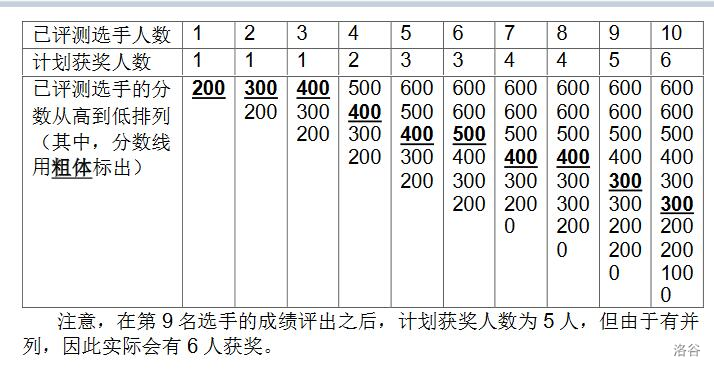### 数据规模与约定

$1 \sim 3$$10$
$4 \sim 6$$500$
$7 \sim 10$$2000$
$11 \sim 17$$10^4$
$18 \sim 20$$10^5$

## 题目分析

1. 每次排名人数 $k_i$ 不是一成不变的，是会变化。
2. 每次输入的分数会导致，排名会发生变化。
3. 数据规模太大。

## 代码实现

#include <iostream>#include <cstdio>using namespace std;const int N=1e5+5;int sc;//sc[x]=x出现的次数int main(){   int n,w,x;   cin>>n>>w;   for(int i=1;i<=n;i++){       cin>>x;       sc[x]++;//统计x出现的次数       int num=max(1,i*w/100);//计算排名，注意最少选一个人       int sum=0;//总人数       for(int j=600;j>=0;j--){//遍历最高分到最低分            sum+=sc[j];//累加该分段的人数            if(sum>=num){//达到排名                cout<<j<<" ";//输出当前分数                break;//结束            }       }   }   return 0;}
]]>
<![CDATA[【题解】gcd区间（ST表）]]> tag:https://www.algorithmnote.cn,2022-05-06:-ti-jie-gcd-qu-jian-st-biao- 2022-05-06T11:33:47+08:00 2022-05-06T11:34:19+08:00 小鱼干 https://www.algorithmnote.cn 题目描述

m次询问，每次询问给定一个区间[L,R]，输出a[L]…a[R]的最大公因数。

## 输入输出样例

5 34 12 3 6 71 32 35 5

137

## 代码实现

#include <iostream>#include <cstdio>using namespace std;const int N=1e3+5;int n,m;int gcd(int a,int b){//辗转相除法求最大公约数while(b){int r=a%b;a=b;b=r;}return a;}int a[N];int Log[N];//Log[x]=log2(x)int st[N][N];//st[i][j]=gcd(i ~ i+(1<<j)-1) int main(){int n,m,l,r;scanf("%d%d",&n,&m);for(int i=1;i<=n;i++){scanf("%d",&a[i]);}//预处理 Log[] 数组Log=0,Log=1;for(int i=3;i<=n;i++){Log[i]=Log[i/2]+1;}//单个元素的最大公约数是自己for(int i=1;i<=n;i++){st[i]=a[i];}//预处理 ST表for(int j=1;(1<<j)<=n;j++){for(int i=1;i+((1<<j)-1)<=n;i++){st[i][j]=gcd(st[i][j-1],st[i+(1<<(j-1))][j-1]);}}while(m--){scanf("%d%d",&l,&r);int len=r-l+1;int Lg=Log[len];//求区间内的最大公约数printf("%d\n",gcd(st[l][Lg],st[r-(1<<Lg)+1][Lg]));}return 0;}
]]>
<![CDATA[【题解】最大子段和]]> tag:https://www.algorithmnote.cn,2022-05-04:-ti-jie--zui-da-zi-duan-he 2022-05-04T14:56:28+08:00 2022-05-06T11:34:33+08:00 小鱼干 https://www.algorithmnote.cn 题目描述

## 输入输出样例

72 -4 3 -1 2 -4 3

4

## 说明/提示

#### 数据规模与约定

• 对于 40% 的数据，保证 $n \leq 2 \times 10^3$
• 对于 100% 的数据，保证 $1 \leq n \leq 2 \times 10^5，-10^4 \leq a_i \leq 10^4$

## 题目分析

1. 该元素与之前的连续区间加起来的和比较大
2. 该元素与之前的连续区间加起来的和还没有这个元素本身大

## 代码实现

#include <iostream>#include <cstdio>using namespace std;int a;/*连续序列和1. 当前元素2. 和前面的一段组成新序列*/int main(){    int n;    int sum=0;//连续序列和    int ans=-1e9;//最大连续序列和    cin>>n;    for(int i=1;i<=n;i++){        cin>>a;        if(sum+a<a){//判断是a与前面的连续序列加起来大还是单独一个元素更大            sum=a;//单独的元素更大        }else{            sum+=a;//加起来更大        }        ans=max(ans,sum);//更新过程中的最大连续序列和    }    cout<<ans;//输出答案    return 0;}
]]>
<![CDATA[【题解】逛画展]]> tag:https://www.algorithmnote.cn,2022-05-03:-ti-jie--guang-hua-zhan 2022-05-03T20:43:55+08:00 2022-05-06T11:34:51+08:00 小鱼干 https://www.algorithmnote.cn 题目描述

Sept 希望入场后可以看到所有名师的图画。当然，他想最小化购买门票的价格。

## 输入输出样例

12 52 5 3 1 3 2 4 1 1 5 4 3

2 7

## 说明/提示

#### 数据规模与约定

• 对于 30% 的数据，有 $n\le200，m\le20$
• 对于 60% 的数据，有 $n\le10^5，m\le10^3$
• 对于 100% 的数据，有 $1\leq n\le10^6，1 \leq a_i \leq m\le2\times10^3$

## 题目解析

1. 区间内不重复的元素个数有m个。
2. 满足条件1的前提下，区间要最小。

## 代码实现

#include <iostream>#include <cstdio>using namespace std;const int N=1e6+5;int a[N];int n,m;int v;//v[x]=画家x在区间内出现的次数int main(){    cin>>n>>m;    for(int i=1;i<=n;i++){        cin>>a[i];    }    int l=1,r=1;//左右指针    int cnt=1;//区间内不同元素个数 ，预处理第一个元素    v[a[l]]=1;//处理第一个元素    int L=1,R=n;//答案区间的左右端点    while(l<n && r<n){//在范围内移动        while(r<n && cnt<m){//右指针移动，直到区间内包含所有的画家(不同元素个数为m)            r++;//移动右指针            v[a[r]]++;//统计出现次数            if(v[a[r]]==1){//判断是否第一次出现                cnt++;//不同元素数量增加            }        }        while(l<=r && cnt==m){//移动左指针            if(r-l<R-L){//若当前满足条件1的区间小于记录的答案区间                //更新区间端点                R=r;                L=l;            }            v[a[l]]--;//统计数量，左指针对应的要从区间内去掉            if(v[a[l]]==0) cnt--;//更新            l++;//移动左指针        }    }    cout<<L<<" "<<R;//输出答案    return 0;}
]]>
<![CDATA[编程办公装机配置]]> tag:https://www.algorithmnote.cn,2022-05-03:bian-cheng-ban-gong-zhuang-ji-pei-zhi 2022-05-03T11:36:17+08:00 2022-05-06T11:35:08+08:00 小鱼干 https://www.algorithmnote.cn 前言

1. 办公。

office 使用。word写教材，excel统计数据。

2. 编程。

C++程序。主要用于刷题。

3. 虚拟机。

开NOI Linux2.0

## 清单如下

### CPU

12代CPU,采用全新架构，性能十分强劲。看了测评，i3-12100 略压十代的i5-10400一筹。而且价格还便宜。因为是用来办公，也不打游戏，故选用核显版本，显卡也不用配了。为了进一步压缩成本，选用淘宝散片。

### 硬盘

M.2借口就上M.2的固态，体验绝对能拉满。挑了下选了致态 TiPlus 1Tb ，速度和价格都很香，读 3500MB/s , 写 3100MB/s ， 寿命600TBW，价格只要 ￥699

### 散热器

CPU散片是不带散热的，所以挑个风冷的散热器就好，这个价位，不会想不开上水冷吧？选择了利民 AX120R SE 不亏是散热器中的卷王，淘宝￥69就能拿下。

### 机箱

CPUi3 12100核显¥744.00淘宝

]]>
<![CDATA[【题解】A-B数对]]> tag:https://www.algorithmnote.cn,2022-05-02:-ti-jie-a-b-shu-dui 2022-05-02T22:25:12+08:00 2022-05-06T11:35:21+08:00 小鱼干 https://www.algorithmnote.cn 题目描述

## 输入输出样例

4 11 1 2 3

3

## 代码实现

#include <iostream>#include <cstdio>#include <algorithm>using namespace std;const int N=2e5+5;int a[N];int n,c;//a-b=c => a-c=bint main(){    cin>>n>>c;    for(int i=1;i<=n;i++){        cin>>a[i];    }    sort(a+1,a+n+1);    int l=1,r=1;    long long sum=0;    for(int i=1;i<=n;i++){        int B=a[i]-c;        while(r<=n &&  a[r]<=B) r++;        while(l<=r && a[l]<B) l++;        sum+=(r-l);    }    cout<<sum;    return 0;}
]]>
<![CDATA[【题解】排列计数]]> tag:https://www.algorithmnote.cn,2022-04-27:-ti-jie--pai-lie-ji-shu 2022-04-27T15:31:25+08:00 2022-04-27T15:31:49+08:00 小鱼干 https://www.algorithmnote.cn 题目描述

## 输入输出样例

51 01 15 2100 5010000 5000

012057802888760695423

## 说明/提示

#### 数据规模与约定

$1\sim 3$$10^3$8$10 \sim 12$$10^3$$10^3$
$4 \sim 6$$10^3$12$13 \sim 14$$5 \times 10^5$$10^3$
$7 \sim 9$$10^3$100$15 \sim 20$$5 \times 10^5$$10^6$

## 题目分析

$C_n^m=\frac{n!}{m!(n-m)!}$

jc=1;jc=1,jc=2;d=0;d=0;d=1;for(int i=3;i<=N-5;i++){    d[i]=(i-1)*(d[i-2]+d[i-1])%M;    jc[i]=jc[i-1]*i%M;}

ll mypow(ll x,ll n,int M){if(n==0) return 1%M;ll tmp=mypow(x,n/2,M)%M;if(n&1) return tmp*tmp%M*x%M;else return tmp*tmp%M;}

## 代码实现

#include <iostream>#include <cstdio>using namespace std;typedef long long ll;const int M=1e9+7;const int N=1e6+5;ll mypow(ll x,ll n){//快速幂 求 x的n次幂if(n==0) return 1%M;ll tmp=mypow(x,n/2)%M;if(n&1) return tmp*tmp%M*x%M;else return tmp*tmp%M;}ll jc[N];//jc[x] = x!ll d[N];//d[x] = x个元素的错排列数ll C(int n,int m){//求组合C(n,m)return jc[n]*mypow(jc[m]*jc[n-m]%M,M-2)%M;}int main(){int t,n,m;scanf("%d",&t);//预处理 阶乘 和 错排列数jc=1;jc=1,jc=2;d=0;d=0;d=1;for(int i=3;i<=N-5;i++){d[i]=(i-1)*(d[i-2]+d[i-1])%M;jc[i]=jc[i-1]*i%M;}while(t--){scanf("%d%d",&n,&m);if(n==m) printf("1\n");//C(n,m)*D(n-m)else printf("%lld\n",C(n,m)*d[n-m]%M);}return 0;}]]>
<![CDATA[乘法逆元]]> tag:https://www.algorithmnote.cn,2022-04-27:cheng-fa-ni-yuan 2022-04-27T15:30:08+08:00 2022-04-27T15:36:34+08:00 小鱼干 https://www.algorithmnote.cn 定义

$a x \equiv 1\ mod \ p$这里$x$就是$a$的逆元。

## 求解逆元的方式

#### 拓展欧几里

$ax \equiv 1\ mod \ m$

gcd(a,b)=1，满足$ax+by=1$,移项得$ax=-by+1$，即$ax\equiv1 \ mod \ b$，此时的x就是逆元。实际上线性不定方程组有无穷多解，这里只求正的最小逆元

ax=-by+1\Rightarrow \left.\begin{aligned}ax \%b&=1 \\1 \ \%b&=1 \\\end{aligned}\right\}\Rightarrowax\equiv1 \ (mod\ b)

int exgcd(int a,int b,int &x,int &y){    if(b==0){        x=1,y=0;        return a;    }    int d=exgcd(b,a%b,x,y);    int z=x;x=y;y=z-y*(a/b);    return d;}ans=(x%b+b)%b;//x可能是负数，需要处理

### 费马小定理

$a^{p-1} \equiv1(mode\ p)$，此时逆元x可取为$a^{p-2}\%p$

### 线性求逆元

$1^{-1}=1,\forall i>=2$,$i^{-1}=(p-\frac{p}{i})\times(p\%i)^{-1}$

$p=k\cdot i+r,r < i,i < i < p$

\left\{\begin{aligned}0 \mod p &=0\\k\cdot i +r \mod p &=0 \end{aligned}\right.\Rightarrow k\cdot i +r\equiv0(\mod p)

$i^{-1}=-k\cdot r^{-1}$

$i^{-1}\equiv-\lfloor \frac{p}{i}\rfloor\cdot(p\mod i)^{-1}(\mod p)$

$dp[i]=i^{-1}$

## 常见用途

$a/b(\mod p)=a\times b^{-1}(\mod p)$

]]>
<![CDATA[错排（错排列）]]> tag:https://www.algorithmnote.cn,2022-04-27:cuo-pai--cuo-pai-lie- 2022-04-27T15:10:52+08:00 2022-04-27T15:10:52+08:00 小鱼干 https://www.algorithmnote.cn 定义

## 递推公式

### 第二步

1. 把它放到位置n
2. 第k个元素不把它放到位置n

\begin{aligned}D(1)&=0\\D(2)&=1\\D(n)&=(n-1)\cdot[D(n-2)+D(n-1)]\end{aligned}

\begin{aligned}D(n)&=n!-\frac{n!}{1!}+\frac{n!}{2!}+\cdots+(-1)^n\times\frac{n!}{n!}\\&=\sum\limits_{k=3}^{n}(-1)^k\cdot\frac{n!}{k!}\end{aligned}

]]>